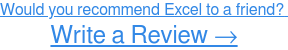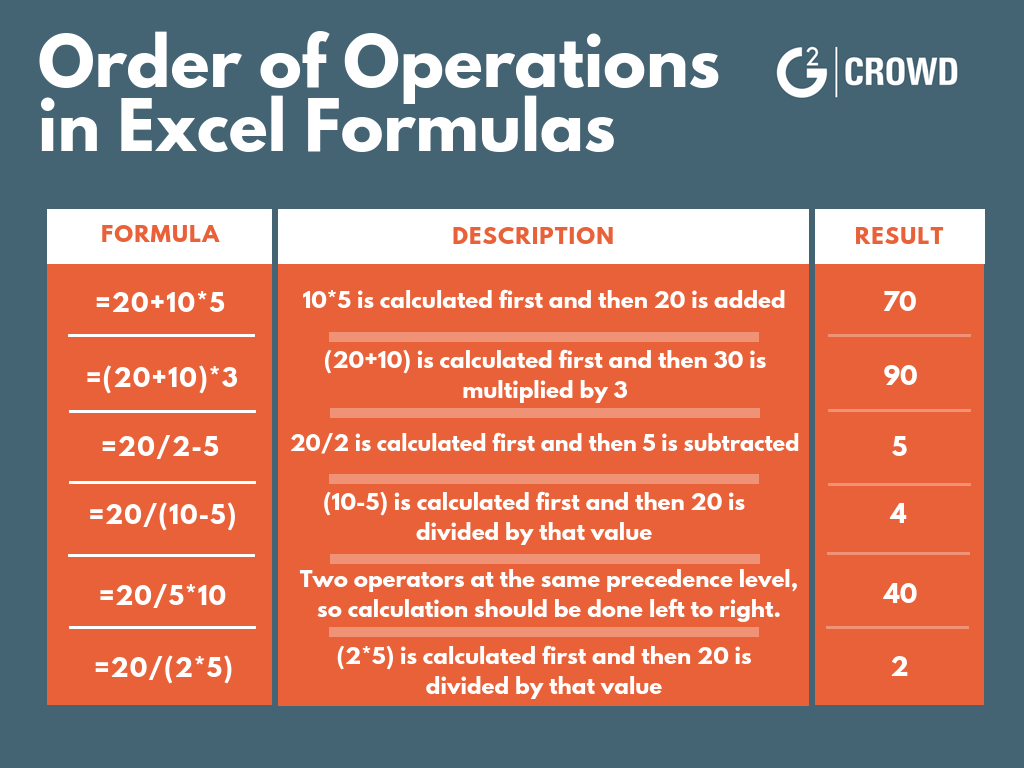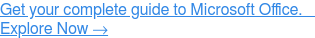Looking to learn how to divide in Microsoft Excel? You’ve come to the right place.

Related: Be sure to check out how to multiply in Excel, how to subtract in Excel, and how to add in Excel# How to divide in Excel

If you’re looking to learn how to divide in Excel, you’ll need to know how to write Excel formulas.

Excel formulas are expressions used to display values that are calculated from the data you’ve entered and they’re generally used to perform various mathematical, statistical, and logical operations.

So before we jump into the specifics of how to divide in Excel, here are a few reminders to keep in mind when writing Excel formulas:

• All Excel formulas should begin with the equal sign (=). This is a must in order for Excel to recognize it as a formula.
• The cells will display the result of the formula, not the actual formula. So by using cell references rather than just entering the data in the cell, if you need to go back later the values will be easier to understand.
• When using arithmetic operators in your formula, remember the order of operations (I personally reference the acronym PEMDAS: Parenthesis, Exponentiation, Multiplication, Division, Addition, Subtraction.) Below you’ll find an example of how the order of operations is applied to formulas in Excel.## How to divide in a cell

To divide numbers in a cell, you'll need to write your formula in the designated cell. Your formula should start with the equal sign (=), and contain the arithmetic operator (/) needed for the calculation. In this case, you want to divide 20 by 2, so your formula is =20/2.Once you’ve entered the formula, press ‘Enter’ and your result will populate. In this example, the division formula, =20/2, yields a result of 10 (see below).## How to divide cells

To divide cells, the data will need to be in its own cells so that you can use the cell references in the formula.

You can see below that a division formula was written in cell C1 using cell references, but the data had to be entered separately in cells A1 and B3.Once you’ve entered the formula, press ‘Enter’ and your result will populate. In this example, the division formula, =A1/B3, yields a result of 5 (see below).## How to divide a range of cells by a constant number

Want to divide each cell in a range by a constant number? By writing your formula with the dollar sign symbol (\$), you’re telling Excel that the reference to the cell is “absolute,” meaning when you copy the formula to different cells, the reference will always be to that cell. In this example, cell C2 is absolute.

That being said, to divide each cell in column A by the constant number in C2, start by writing your formula as you would if you were dividing cells, but add ‘\$’ in front of the column letter and row number. In this case, your formula is =A2/\$C\$2.Next, drag the formula to the other cells in the range. This copies the formula to the new cells, updating the data, but keeping the constant.

The results will populate as you drag and drop the formula (see below).## How to use the QUOTIENT function

With division, sometimes results will have a remainder. For example, if you enter the multiplication formula =10/6 in Excel, your result would be 1.6667.

The QUOTIENT function, though, will only return the integer portion of a division – not the remainder. Therefore, you should use this function if you want to discard of the remainder.

To use this function, navigate to the ‘Formulas’ tab and click ‘Insert Function’ or start typing ‘=QUOTIENT’ in the cell you want the result to populate in. Select ‘QUOTIENT.’From there, enter your cell references (or numbers) in the function. Be sure to separate the cell references with a comma (,).Press ‘Enter’ and the integer result – without the remainder – will populate. So, rather than =10/6 equaling 1.6667, using the QUOTIENT function yields 1.You’re ready to divide in Excel!

And that’s it! In this article, we’ve covered everything you need to know about how to divide in Excel using formulas and functions.

Now grab your computer and dive into Excel to give it a try for yourself!

Did you enjoy this article? Check out our articles on how merge cells in Excel and how to lock cells in Excel!Never miss a post.

Subscribe to keep your fingers on the tech pulse.